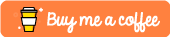Python Multiprocessing Tutorial

Last Updated: 1st December, 2018

Introduction

Previously we’ve looked at how you can try to achieve concurrency with Python using multithreading, the tutorial of which can be found here: Python Multithreading Tutorial

Now we are going to be having a look at how we can sidestep the limiting Global Interpreter Lock that effectively prevented our multithreaded applications from being truly concurrent by using the multiprocessing Python module.

Parallelism vs Concurrency

So, before we go deeper into the multiprocessing module, it’s worthwhile ensuring you know the advantages of using multiprocessing over multithreading. The general rule of thumb is that, if you are trying to improve the performance of CPU-bound tasks, multiprocessing is what you want to use. However, if your particular task is Input/Output bound, then you’ll generally want to use multithreading to improve the performance of your applications.

A Simple Example:

Let’s start by building a really simple Python program that utilizes the multiprocessing module.

In this example, I’ll be showing you how to spawn multiple processes at once and each process will output the random number that they will compute using the random module.

from multiprocessing import Process, Queue
import random

def rand_num():
num = random.random()
print(num)

if __name__ == "__main__":
queue = Queue()

processes = [Process(target=rand_num, args=()) for x in range(4)]

for p in processes:
p.start()

for p in processes:
p.join()

Running this should then print out an array of 4 different decimal numbers between 0 and 1 like so:

Elliots-MacBook-Pro:Networking elliotforbes\$ python processing.py
0.090906474002
0.306163229303
0.995446921388
0.0320995066016

Now, the important thing to note here, is that each of these random numbers was generated in an entirely separate Python process created with the help of the multiprocessing module.

Each of these separate processes features it’s own instance of the Global Interpreter Lock, and each of these can be run across multiple CPU cores. Now, let’s imagine we were doing something more CPU-intensive than simply generating a single random number. This is where the multiprocessing module would truly start to shine.

Pools

In the previous example, we looked at how we could spin up individual processes, this might be good for a run-and-done type of application, but when it comes to longer running applications, it is better to create a pool of longer running processes. This avoids having to create and destroy a process every time you have a new task to execute and can subsequently improve the performance of your applications.

import multiprocessing as mp

def my_func(x):
print(x**x)

def main():
pool = mp.Pool(mp.cpu_count())
result = pool.map(my_func, [4,2,3])

if __name__ == "__main__":
main()

Now, if we were to execute this, we’d see our my_func being executed with the array [4,2,3] being mapped as the input to each of these function calls.

python3.6 main.py
256
4
27

Let’s expand this just a little bit to showcase some key concepts here:

import multiprocessing as mp

def my_func(x):
print(mp.current_process())
return x**x

def main():
pool = mp.Pool(mp.cpu_count())
result = pool.map(my_func, [4,2,3,5,3,2,1,2])
result_set_2 = pool.map(my_func, [4,6,5,4,6,3,23,4,6])

print(result)
print(result_set_2)

if __name__ == "__main__":
main()

So, we’ve added a second print statement to the my_func function. This is to highlight what process within our pool will be executing a particular task. We’ve also changed from a print(x**x) to a return x**x so that we can view the results of our process pool.

Within our main() function, we’ve added a few more values to the array in a map so that we can truly test the extent of our process pool’s workers. We’re also printing out the results which should now be populated thanks to our newly added returns in our my_func.

When we execute this, we should see the following output:

\$ python3.6 main.py
256
<ForkProcess(ForkPoolWorker-1, started daemon)>
4
<ForkProcess(ForkPoolWorker-2, started daemon)>
27
...
20880467999847912034355032910567
<ForkProcess(ForkPoolWorker-6, started daemon)>
<ForkProcess(ForkPoolWorker-7, started daemon)>
256
46656
<ForkProcess(ForkPoolWorker-2, started daemon)>
<ForkProcess(ForkPoolWorker-8, started daemon)>
[256, 4, 27, 3125, 27, 4, 1, 4]
[256, 46656, 3125, 256, 46656, 27, 20880467999847912034355032910567, 256, 46656]

As you can see, our each job is executed by one of the 8 workers that exist within the pool. These processes are reused in order to prevent the costly task of destroying and creating new processes and subsequent execution.

Passing Data Between Processes

When performing concurrent programming the best practice is usually to avoid sharing state as much as possible. However when you absolutely need to have some form of shared data then the multiprocessing module provides a couple of ways of doing so.

Queues:

Queue objects are a FIFO data structure that are thread and process safe which make them perfect for passing data between different processes without potentially corrupting data. Using them is relatively simple, we can expand:

from multiprocessing import Process, Queue
import random

def rand_num(queue):
num = random.random()
queue.put(num)

if __name__ == "__main__":
queue = Queue()

processes = [Process(target=rand_num, args=(queue,)) for x in range(4)]

for p in processes:
p.start()

for p in processes:
p.join()

results = [queue.get() for p in processes]

print(results)

This should output the following:

Elliots-MacBook-Pro:Networking elliotforbes\$ python processing.py
[0.6756465745753756, 0.4104274331895341, 0.6733748532075048, 0.9494118991646461]

Conclusion

Overall Python’s MultiProcessing module is brilliant for those of you wishing to sidestep the limitations of the Global Interpreter Lock that hampers the performance of the multi-threading in python.

I hope this has been helpful, if you feel anything else needs added to this tutorial then let me know in the comments section below! Also, feel free to follow me on twitter for up-to-date announcements and all the latest programming articles: @Elliot_f.